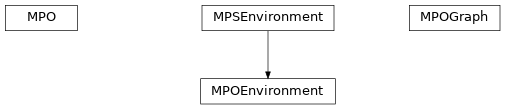# mpo¶

ClassesMPO(sites, Ws[, bc, IdL, IdR, max_range, …]) Matrix product operator, finite (MPO) or infinite (iMPO). MPOEnvironment(bra, H, ket[, init_LP, …]) Stores partial contractions of  for an MPO H. MPOGraph(sites[, bc, max_range]) Representation of an MPO by a graph, based on a ‘finite state machine’.

Functions

 grid_insert_ops(site, grid) Replaces entries representing operators in a grid of W[i] with npc.Arrays. make_W_II(t, A, B, C, D) W_II approx to exp(t H) from MPO parts (A, B, C, D).

Module description

Matrix product operator (MPO).

An MPO is the generalization of an MPS to operators. Graphically:

|      ^        ^        ^
|      |        |        |
|  ->- W ->- W ->- W ->- ...
|      |        |        |
|      ^        ^        ^


So each ‘matrix’ has two physical legs p, p* instead of just one, i.e. the entries of the ‘matrices’ are local operators. Valid boundary conditions of an MPO are the same as for an MPS (i.e. 'finite' | 'segment' | 'infinite'). (In general, you can view the MPO as an MPS with larger physical space and bring it into canoncial form. However, unlike for an MPS, this doesn’t simplify calculations. Thus, an MPO has no form.)

We use the following label convention for the W (where arrows indicate qconj):

|            p*
|            ^
|            |
|     wL ->- W ->- wR
|            |
|            ^
|            p


If an MPO describes a sum of local terms (e.g. most Hamiltonians), some bond indices correspond to ‘only identities to the left/right’. We store these indices in IdL and IdR (if there are such indices).

Similar as for the MPS, a bond index i is left of site i, i.e. between sites i-1 and i.